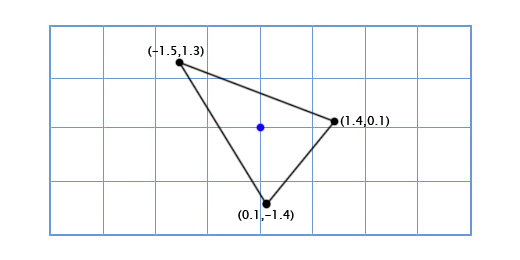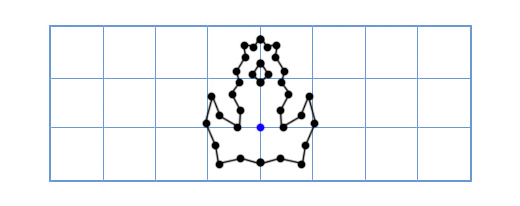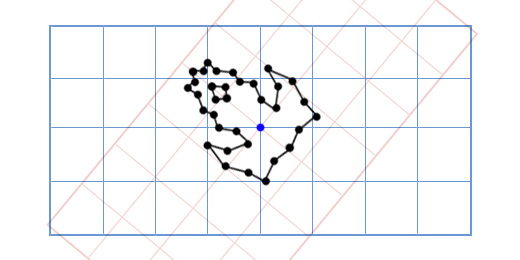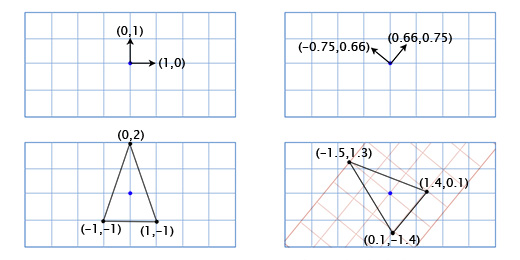# Linear algebra for game developers ~ part 3

July 21st, 2010

I introduced vectors in Part 1, and a toolset for working with them in Part 2. However, today I would like to talk about perhaps the most important concept for game developers. This is the concept of the transformation matrix.

Let's start by looking at the building blocks of the transformation matrix:

## Basis Vectors

Let's say we're making an Asteroids game on really old hardware, and we want to have a simple 2D space ship that can rotate freely. The ship model looks like this:So how do we draw it when the player rotates by an arbitrary amount, like 49 degrees counter-clockwise? Well, trigonometry we can create a function for 2D rotation that inputs a point and an angle, and outputs a rotated point:

```   vec2 rotate(vec2 point, float angle){
vec2 rotated_point;
rotated_point.x = point.x * cos(angle) - point.y * sin(angle);
rotated_point.y = point.x * sin(angle) + point.y * cos(angle);
return rotated_point;
}
```
Applying this to our three points gives us the following shape:Cosine and sine operations are pretty slow, but we're only doing them on three points, so it can smoothly on our old hardware. But now we decide to upgrade the ship to look like this:Now our old method is too slow! We don't have enough sine and cosine operations to rotate this many points. There are many ways to solve this problem, but an elegant solution comes to us like this: "What if instead of rotating each point in the model, we just rotate the model's x and y axes instead?"How does this work? Well, let's look at what coordinates mean. When we talk about the the point (3,2), we are saying that its position is three times the x-axis plus two times the y-axis. The default axes are (1,0) for the x-axis and (0,1) for the y-axis, so we get the position 3(1,0) + 2(0,1). But the axes don't have to be (1,0) and (0,1). If we rotate these axes, then we can rotate every point at the same time.

To get the rotated x and y axes we just use the trigonometric function above. For example, if we are rotating by 49 degrees, then we get the new x-axis by rotating (1,0) by 49 degrees and we get the y-axis by rotating (0,1) by 49 degrees. Our new x-axis is (0.66, 0.75), and our new y-axis is (-0.75, 0.66). We can do this by hand for our 3-point model to prove that it works:

The top point is (0,2), which means its new position is zero times the rotated x-axis plus twice the rotated y-axis.

0*(0.66,0.75) + 2*(-0.75, 0.66) = (-1.5, 1.3)

The bottom-left point is (-1,-1), which means its new position is negative one times the rotated x-axis plus negative one times the rotated y-axis.

-1*(0.66,0.75) + -1*(-0.75, 0.66) = (0.1, -1.4)

The bottom-right point is (1,-1), which means its new position is one times the rotated x-axis plus negative one times the rotated y-axis.

1*(0.66,0.75) + -1*(-0.75, 0.66) = (1.4, 0.1)What we are doing here is using the ship coordinates as if they exist in a separate coordinate system with rotated axes (or 'basis vectors'). This is useful in this case because it separates the cost of manipulating the orientation of the ship from the cost of applying the orientation to the model points.

Whenever you have modified the basis vectors (1,0) and (0,1) to (a,b) and (c,d), then the modified point (x,y) can be found using this expression:

x(a,b) + y(c,d)

Normally the basis vectors are (1,0) and (0,1) so you just get x(1,0) + y(0,1) = (x,y), and you don't have to bother with this. However, it's important to remember that you can use other bases (plural of basis) as well.

## Matrices

Matrices are like 2-dimensional vectors. For example, a typical 2x2 matrix might look like this:

```   [a c
b d]
```

When you multiply a matrix by a vector, you sum the dot product of each row of the matrix with the vector. For example, if we multiply the above matrix with the vector (x,y), we get:

(a,c)•(x,y) + (b,d)•(x,y)

Written another way, this is:

x(a,b) + y(c,d)

Look familiar? This is the exact same expression that we use when changing the basis vectors! This means that multiplying a 2x2 matrix with a 2D vector is the same thing as changing its basis vectors. For example, if we plug the standard basis vectors (1,0) and (0,1) into the matrix columns, we get:

```[1 0
0 1]
```

This is the identity matrix, which has no effect, as we would expect from the neutral basis vectors we chose. If we plug in our 49-degree rotation bases, we get:

```[0.66 -0.75
0.75  0.66]
```

This matrix will rotate any 2D vector counter-clockwise by 49 degrees. We could write our asteroids program with a lot more elegance by using matrices like this. For example, our ship-rotation function could look like this:

```void RotateShip(float degrees){
Matrix2x2 R = GetRotationMatrix(degrees);
for(int i=0; i<num_points; ++i){
rotated_point[i] = R * point[i];
}
}
```
However, it would be even more elegant if we could include translation (movement through space) in the matrix as well. Then we would have a single data structure that can elegantly store and apply the orientation and position of each object. Fortunately, there is a way to do this, even though it's a bit of an ugly hack. If we want to translate by a vector (e,f), we can just tack it onto our change-of-basis matrix like this:

```[a c e
b d f
0 0 1]
```

And then we add an extra  to the end of each position vector like this:

[x y 1]

Now when we multiply them together we get:

(a,c,e)•(x,y,1) + (b,d,f)•(x,y,1) + (0,0,1)•(x,y,1)

Which can essentially be rewritten:

x(a,b) + y(c,d) + (e,f)

So now we have the entire transformation in a single matrix! This is important for many reasons aside from just elegant code, because now we can use all of the standard matrix manipulation tools on it. For example, we can multiply matrices together to add their effects, or we can invert a matrix to give it the exact opposite transformation.

## 3D Matrices

Matrices in 3D work just like they do in 2D -- I just used 2D examples in this post because they are easier to convey with a 2D screen. You just define three columns for the basis vectors instead of two. If the basis vectors are (a,b,c), (d,e,f) and (g,h,i) then your matrix should be:

```[a d g
b e h
c f i]
```

If you need translation (j,k,l), then you add the extra column and row like before:

```[a d g j
b e h k
c f i l
0 0 0 1]
```

And add an extra  onto the vectors like this:

[x y z 1]

There is a lot more to say about matrix transformations, but I thought I should stop here to see if this makes any sense. Do you have any questions about this topic, or any ideas for future ones? Here is part 4, about 3D rotations!